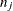# The PRINCOMP Procedure

### FREQ Statement

`FREQ` variable ;

The FREQ statement specifies a variable that provides frequencies for each observation in the DATA= data set. Specifically, if n is the value of the FREQ variable for a given observation, then that observation is used n times.

The analysis produced using a FREQ statement reflects the expanded number of observations. The total number of observations is considered equal to the sum of the FREQ variable. You could produce the same analysis (without the FREQ statement) by first creating a new data set that contains the expanded number of observations. For example, if the value of the FREQ variable is 5 for the first observation, the first 5 observations in the new data set would be identical. Each observation in the old data set would be replicatedtimes in the new data set, whereis the value of the FREQ variable for that observation.

If the value of the FREQ variable is missing or is less than one, the observation is not used in the analysis. If the value is not an integer, only the integer portion is used.